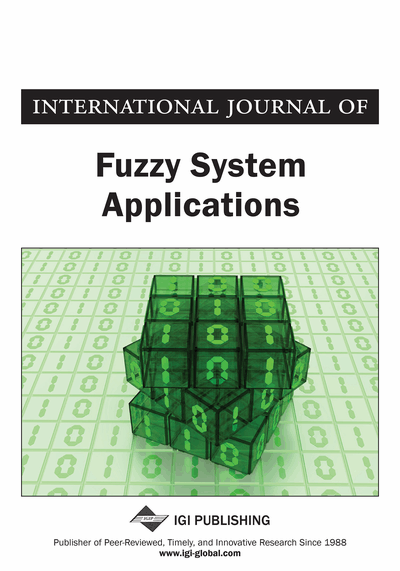# Group Decision Making Through Interval Valued Intuitionistic Fuzzy Soft Sets

B. K. Tripathy (VIT University, Vellore, India), T. R. Sooraj (Providence College of Engineering, Chengannur, Kerala, India), R. K. Mohanty (VIT University, Vellore, India) and Abhilash Panigrahi (VIT University, Vellore, India)
DOI: 10.4018/IJFSA.2018070106
Available
\$37.50
No Current Special Offers

## Abstract

This article describes how the lack of adequate parametrization in some of the earlier uncertainty based models like fuzzy sets, rough sets motivated Molodtsov to introduce a new model in soft set. A suitable combination of individual models leads to hybrid models, which are more efficient than their individual components. So, the authors find the introduction of many hybrid models of soft sets, like the fuzzy soft set (FSS), intuitionistic fuzzy soft sets (IFSS), interval valued fuzzy soft set (IVFSS) and the interval valued intuitionistic fuzzy soft set (IVIFSS). Following the characteristic function approach to define soft sets introduced by Tripathy et al., they re-define IVIFSS in this article. One of the most attractive applications of soft set theory and its hybrid models has been decision making in the form of individual decision making or group decision making. Here, the authors propose a group decision making algorithm using IVIFSS, which generalises many of our earlier algorithms. They compute its complexity and establish the computation experimentally with graphical illustrations.
Article Preview
Top

## Introduction

In real life, we come across uncertain databases more frequently. As a result, several uncertainty-based models are found in literature to handle these data sets. Notable among them are fuzzy sets by Zadeh (1965), rough sets by Pawlak (1982). Molodtsov (1999) observed that these models lack sufficient parametrization and so he introduced a new model called soft sets, which is parameterized family of subsets defined over a universe. Parameter can be words, sentences, real numbers, phrases etc. Later in 2015, Tripathy et al (2015) used characteristic functions to define soft sets. This approach provides rigour to proofs of properties of soft sets and makes it easier to define operations on them. Some applications of soft sets are discussed by Molodtsov (1999). Maji et al. (2001) discussed an application of soft sets in decision making problems. Tripathy et al. (2016a, 2016b) introduced the concept of negative parameters and incorporated the use of positive and negative parameters in decision making applications. As an extension of fuzzy sets, intuitionistic fuzzy sets were introduced by Atanassov in 1986. It generalised the non-membership function and thereby introduced a hesitation function. Since hybrid models provide better results than the individual ones, many hybrid models involving soft sets have been introduced. Following it fuzzy soft sets were introduced in Maji et al (2001a) and redefined in Tripathy et al (2016a). Maji et al. (2001b) introduced intuitionistic fuzzy soft sets and also discussed a decision-making application. Tripathy et al (2016b, 2016d) redefined intuitionistic fuzzy soft sets and improved the algorithm by Maji et al. (2001) with the help of negative parameters. Interval valued fuzzy sets are another extended version of fuzzy sets. Yang et al. (2009) introduced the hybrid model of interval valued fuzzy soft sets (IVFSS) and discussed on decision making using IVFSS. Also, IVFSS was redefined by Tripathy et al. (2016a) and an algorithm for decision making was proposed. IVFSS was extended further by Jiang et al. (2010) to introduce interval valued intuitionistic fuzzy soft sets (IVIFSS). They discussed some properties of IVIFSS and decision-making application of IVIFSS.

Several group decision-making algorithms are found in the literature (Deng Feng Li 2014, 2016; Deng Feng Li et al, 2010a, b; Wan et al., 2015). We find such algorithms in Sooraj et al. (2016) for FSS, Tripathy et al (2016c, 2016e) for IFSS and Tripathy et al (2015) for IVFSS. They mentioned the impact of negative parameters which adversely affects the decision-making process (Tripathy et al., 2016a, 2016b, 2016c, 2016d, 2016e, 2016f, 2016g, 2018; 2017; Sooraj et al., 2017a, 2017b; Mohanty et al., 2017). In this paper, we re-define IVIFSS using the membership and non-membership functions approach, define some operations on them and put forth an algorithm for group decision making using IVIFSS. Most importantly, we compute the complexity of this algorithm empirically and verify it through experimentation. The experimental results are presented graphically.

## Complete Article List

Search this Journal:
Reset
Open Access Articles: Forthcoming
Volume 11: 4 Issues (2022): Forthcoming, Available for Pre-Order
Volume 10: 4 Issues (2021): 3 Released, 1 Forthcoming
Volume 9: 4 Issues (2020)
Volume 8: 4 Issues (2019)
Volume 7: 4 Issues (2018)
Volume 6: 4 Issues (2017)
Volume 5: 4 Issues (2016)
Volume 4: 4 Issues (2015)
Volume 3: 4 Issues (2013)
Volume 2: 4 Issues (2012)
Volume 1: 4 Issues (2011)
View Complete Journal Contents Listing